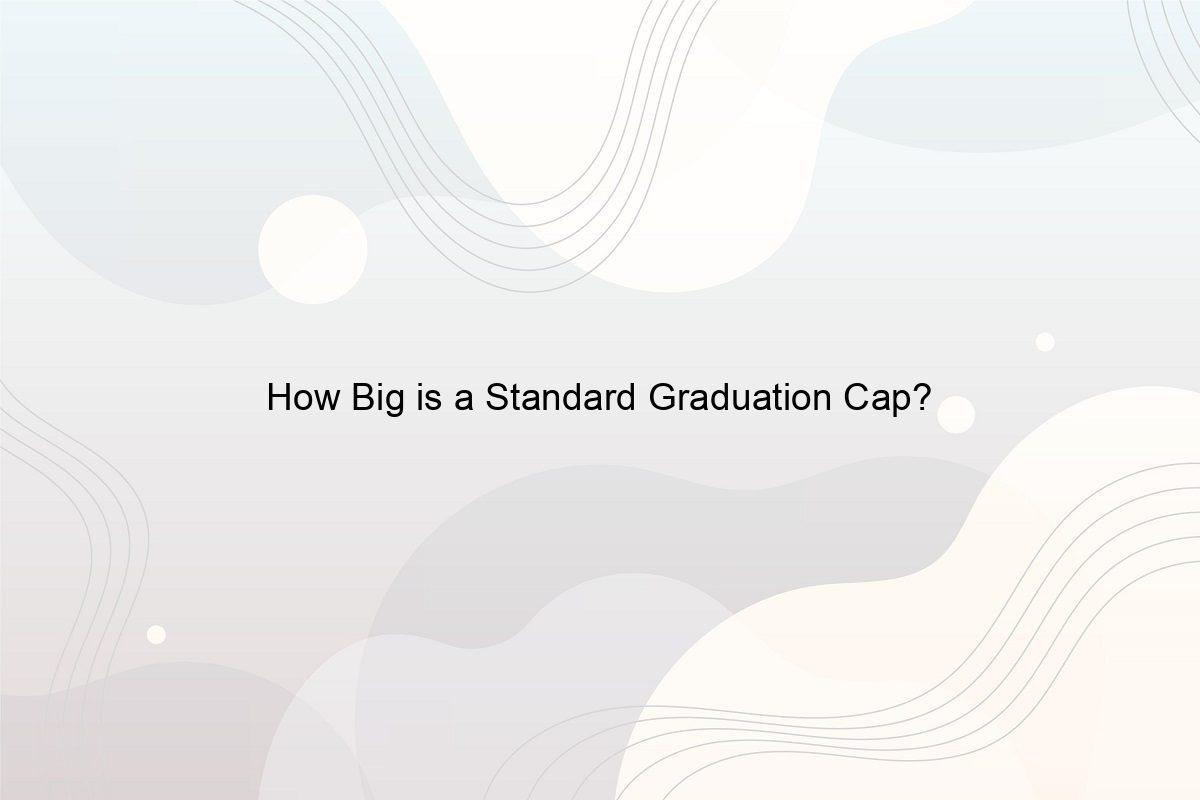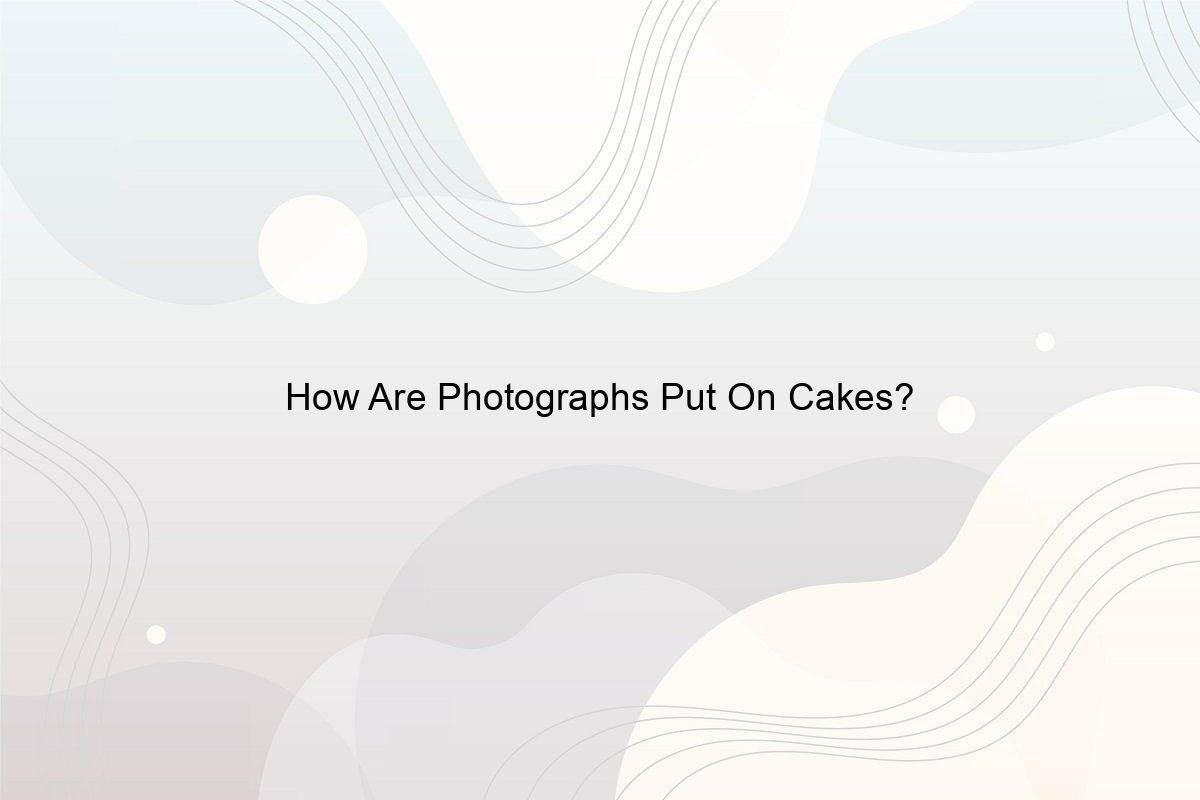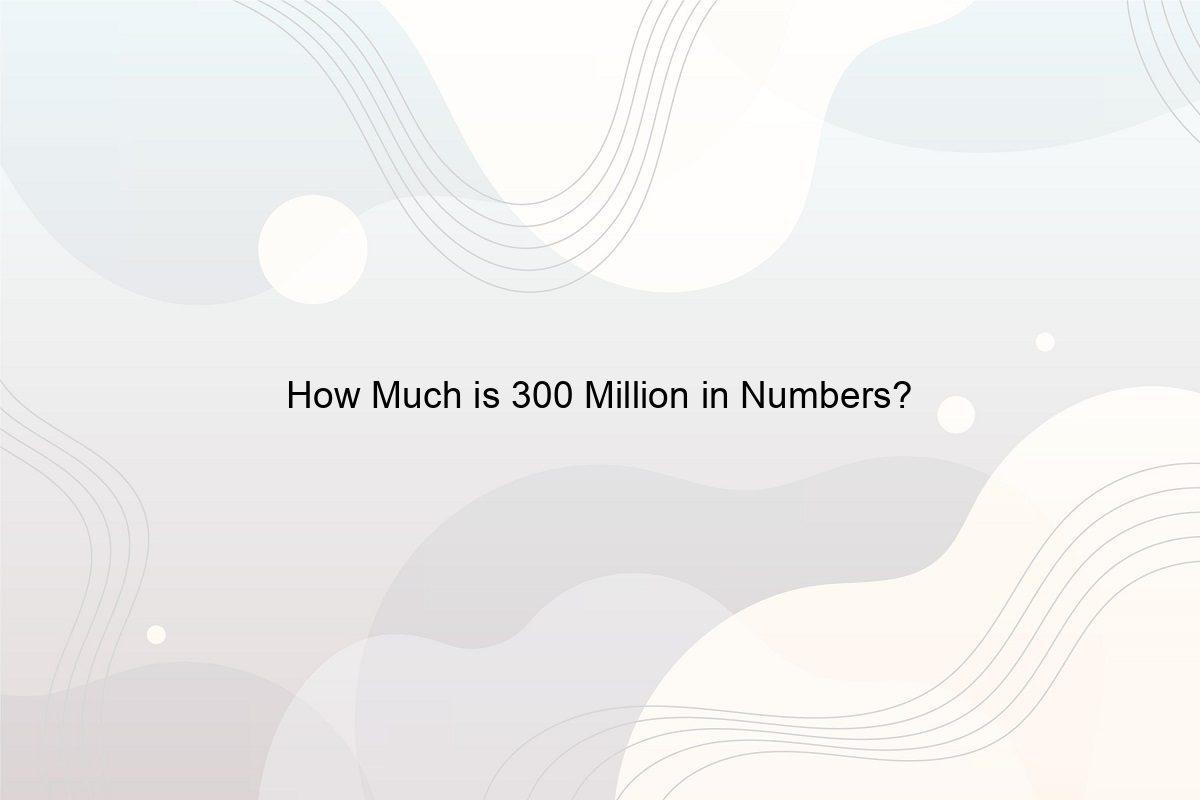﻿ What is a Mathematical Sentence Example? - Speeli

# What is a Mathematical Sentence Example?

What is a Mathematical Sentence? How can You write a Mathematical Sentence? What is the Mathematical Equation?

Mathematical sentences, like English phrases, serve to represent information in the real world. However, they employ their language in a manner that involves operations and variables. They are made up of mathematical formulas or number sentence for multiplication and other operations that include symbols for equality (=) and inequality (≠, >, <) to define a whole concept. Today in this article you will get to know about mathematical sentence example along with mathematical equation and a lot more things. So, continue reading the article.

### 1. What is a Mathematical Sentence?

A mathematical sentence consists of two expressions and makes a statement about them. Both of these expressions make use of either an integer, variables, or a combination of the two types of elements. The sentence ultimately establishes some relations between them using equality and non-equality symbols. (See Who Invented Math?)

### 2. How can You write a Mathematical Sentence?

We can write any number-relation statements in terms of mathematical expressions. For example, I have three more shirts than my roommate is an English sentence.

So, let’s assume that my roommate had an S number of shirts and that I have an M number of shirts. If I said that I have three more shirts than she had, then it would suggest that her clothes added up to my shirts, which mathematically might be represented as S plus 3 equals M. The expression will be S + 3 = M. (See What does 2/3 plus 2/3 equal?)

### 3. What is a Mathematical Sentence Example?

photo by Gerd Altmann on Pixabay

A mathematical sentence example is given below

Mr. neighborhood’s class had 23 boys and girls. Let’s take B and G as variables for boys and girls. B is referring to the number of boys and G represents the number of girls. So, if we say that B + G = 23, it means that the number of boys and the number of girls is equal to 23.

Another mathematical sentence example is, B = G − 3, which tells us that the number of boys is three less than the number of girls. Although it’s kind of a different mathematical grammar is followed in mathematics rather than the use in proper English grammar. Also, check out why is mathematical concept important?

### 4. What is the Mathematical Equation?

How do we write three plus seven times a number in mathematics and what is that number if the total sum equals 10?

Let’s suppose that number is x. And the equation  is 7x + 3 = 10,

Then, 7x = 10 − 3 or 7x = 7

Thus, x = 1 is the required number.

### 5. What are the Two Types of Mathematical Sentences?

Sentences that give us information are called mathematical sentences and there are two types of mathematical sentences open sentences and closed sentences. A closing statement tells us the exact accuracy of a sentence by stating whether it’s true or false which is not the case for unopened sentences as it uses variables and does not tell whether the sentence is true or false. Also, check out what are the types of functions?

### 6. When can we say that a Mathematical Sentence is True?

When a sentence professes the reflexive property of mathematics that is if x = x, and it follows the laws of logic and proves what it asserts is the case. For example, 2 + 2 = 4 is true because by adding 2 and 2 we get a four.

Another example could be the train coming 15 minutes late every day after assessing that it does come 15 minutes late will be a true sentence. So, for a sentence to be true, one side of the equality symbol should be equal to the other side. (See How to Write 1 Million in Numbers?)

### 7. What is a Number Sentence in Math?

Photo by Alicja on Pixabay

A number sentence is an equation made up of numbers, operation symbols like addition, subtraction, multiplication, or division, and an equality symbol. (See When Were Numbers invented?)

### 8. How can You write a Number Sentence for Multiplication?

Mathematical action words used in number sentence for multiplication are multiply by, each, per, groups of, by, a product of, by lots of, equal groups, times, and as much.

In a multiplication number sentence, 2 × 3 = 6 here, 2 is the multiplicand and 3 is a multiplier, and 6 is the product. Multiplicand times multiplier equals product is the correct sequence of writing a multiplication sentence. Also, check out what are some examples of categorical variables?

### 9. Is Number Sentence and Equation the Same?

Photo by Antoine Dautry on Unsplash

A number sentence requires:

• at least two numbers,
• an inequality,
• an operation, and

An equation as well needs at least two numbers, operation equality, and a variable that should be unknown. Must read what is the very last number in the world?

### 10. What is a Mathematical Sentence in One Variable of Degree 2?

A quadratic equation with one variable is a mathematical expression of degree 2. It can be represented in its standard form of mathematical equation as ax² + bx + c = 0, where a, b, and c are real numbers, and x is the variable.

Few examples of a mathematical sentence in quadratic equations are, 2x² – 4x – 2 = 0 and x² – 5x – 6 = 0. (Must read What Is Algebra Used For In Real Life?)

##### Related Posts## How Big is a Standard Graduation Cap?

How Big is a Standard Graduation Cap? How Big is the Circle on a Graduation Cap? Are all Graduation Caps the Same Size?## How Are Photographs Put On Cakes?

Can we eat photo cake? These special inks are made from sugar and food colouring. Most edible photos have very little texture and no significant taste.